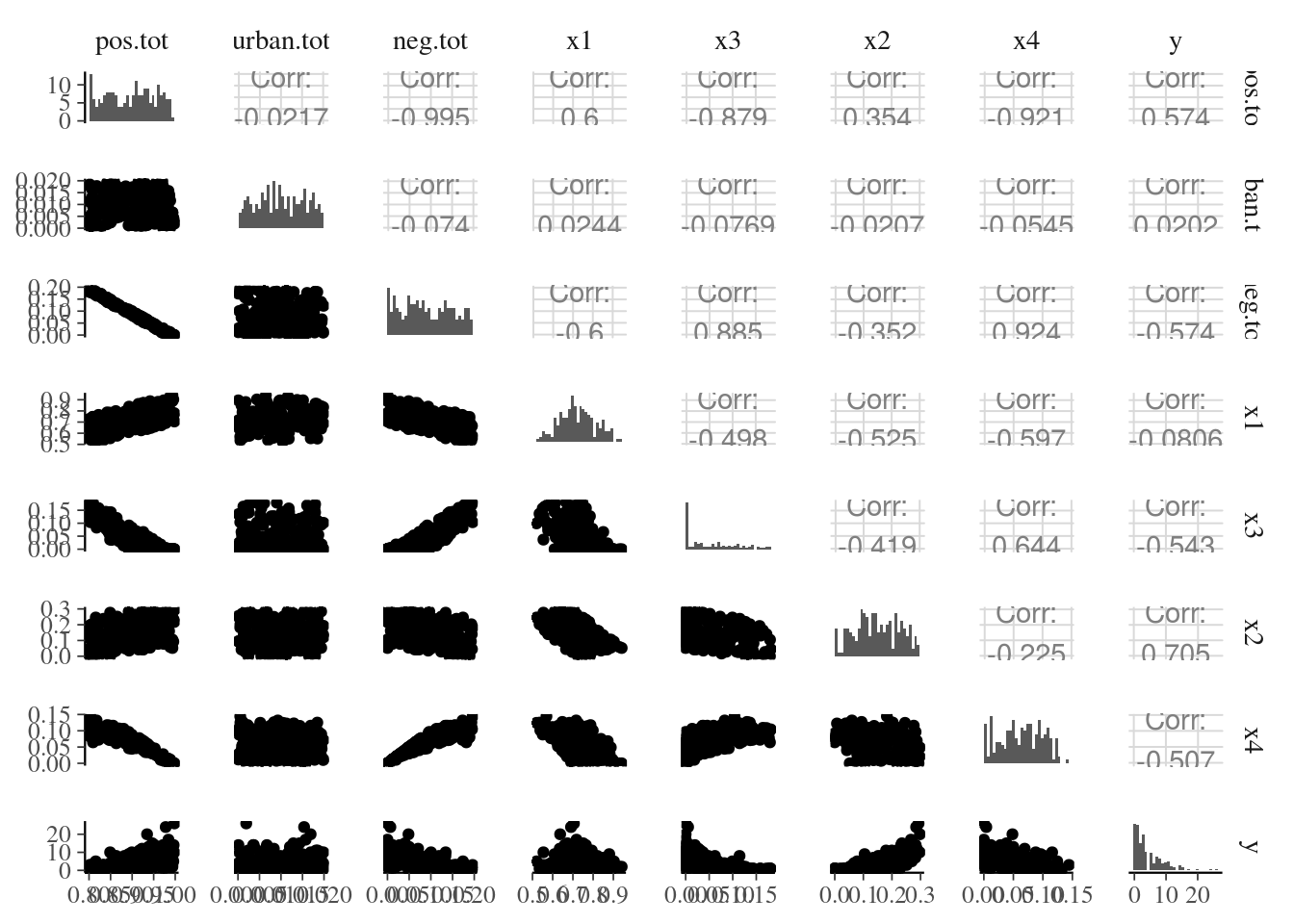# Setup

``````library(rstanarm)
options(mc.cores = parallel::detectCores())
library(loo)
library(tidyverse)
library(GGally)
library(bayesplot)
theme_set(bayesplot::theme_default())
library(projpred)
SEED=87``````

# 1 Introduction

This notebook was inspired by Andrew Tyre’s blog post Does model averaging make sense?. Tyre discusses problems in current statistical practices in ecology, focusing in multi-collinearity, model averaging and measuring the relative importance of variables. Tyre’s post is commenting a paper Model averaging and muddled multimodel inferences In his blog post he uses maximum likelihood and AIC_c. Here we provide a Bayesian approach for handling multicollinearity, model averaging and measuring relative importance of variables using packages `rstanarm`, `bayesplot`, `loo` and `projpred`. We demonstrate the benefits of Bayesian posterior analysis (Gelman et al., 2013) and projection predictive approach (Piironen and Vehtari, 2017; Piironen, Paasiniemi and Vehtari, 2018).

# 2 Data

We generate the data used previously to illustrate multi-collinearity problems.

``````# all this data generation is from Cade 2015
# doesn't matter what this is -- if you use a different number your results will be different from mine.
set.seed(SEED)
df <- tibble(
pos.tot = runif(200,min=0.8,max=1.0),
urban.tot = pmin(runif(200,min=0.0,max=0.02),1.0 - pos.tot),
neg.tot = (1.0 - pmin(pos.tot + urban.tot,1)),
x1= pmax(pos.tot - runif(200,min=0.05,max=0.30),0),
x3= pmax(neg.tot - runif(200,min=0.0,max=0.10),0),
x2= pmax(pos.tot - x1 - x3/2,0),
x4= pmax(1 - x1 - x2 - x3 - urban.tot,0))
# true model and 200 Poisson observations
mean.y <- exp(-5.8 + 6.3*df\$x1 + 15.2*df\$x2)
df\$y <- rpois(200,mean.y)

ggpairs(df,diag=list(continuous="barDiag"))``````Tyre: “So there is a near perfect negative correlation between the things sage grouse like and the things they don’t like, although it gets less bad when considering the individual covariates.”

# 3 Bayesian inference

From this point onwards we switch to Bayesian approach. The rstanarm package provides `stan_glm` function which accepts same arguments as `glm`, but makes full Bayesian inference using Stan (mc-stan.org). By default a weakly informative Gaussian prior is used for weights.

``fitg <- stan_glm(y ~ x1 + x2 + x3 + x4, data = df, na.action = na.fail, family=poisson(), seed=SEED)``

Let’s look at the summary:

``summary(fitg)``
``````
Model Info:
function:     stan_glm
family:       poisson [log]
formula:      y ~ x1 + x2 + x3 + x4
algorithm:    sampling
sample:       4000 (posterior sample size)
priors:       see help('prior_summary')
observations: 200
predictors:   5

Estimates:
mean   sd    10%   50%   90%
(Intercept)   2.7    5.6  -4.1   2.6   9.8
x1           -2.2    5.7  -9.4  -2.1   4.7
x2            6.3    5.7  -0.9   6.4  13.2
x3           -9.1    5.7 -16.4  -9.0  -1.9
x4           -8.9    6.0 -16.6  -8.8  -1.7

Fit Diagnostics:
mean   sd   10%   50%   90%
mean_PPD 4.3    0.2  4.1   4.3   4.6

The mean_ppd is the sample average posterior predictive distribution of the outcome variable (for details see help('summary.stanreg')).

MCMC diagnostics
mcse Rhat n_eff
(Intercept)   0.2  1.0   770
x1            0.2  1.0   770
x2            0.2  1.0   773
x3            0.2  1.0   805
x4            0.2  1.0   792
mean_PPD      0.0  1.0  2471
log-posterior 0.0  1.0  1225

For each parameter, mcse is Monte Carlo standard error, n_eff is a crude measure of effective sample size, and Rhat is the potential scale reduction factor on split chains (at convergence Rhat=1).``````

We didn’t get divergences, Rhat’s are less than 1.1 and n_eff’s are useful (see, e.g., RStan workflow). However, when we know that covariats are correlating we can get even better performance by using QR decomposition (see, The QR Decomposition For Regression Models).

``fitg <- stan_glm(y ~ x1 + x2 + x3 + x4, data = df, na.action = na.fail, family=poisson(), QR=TRUE, seed=SEED)``

Let’s look at the summary and plot:

``summary(fitg)``
``````
Model Info:
function:     stan_glm
family:       poisson [log]
formula:      y ~ x1 + x2 + x3 + x4
algorithm:    sampling
sample:       4000 (posterior sample size)
priors:       see help('prior_summary')
observations: 200
predictors:   5

Estimates:
mean   sd    10%   50%   90%
(Intercept)   2.6    6.0  -5.1   2.7  10.2
x1           -2.1    6.1  -9.8  -2.2   5.7
x2            6.4    6.1  -1.3   6.3  14.2
x3           -9.0    6.0 -16.6  -9.0  -1.2
x4           -8.8    6.4 -16.9  -8.9  -0.7

Fit Diagnostics:
mean   sd   10%   50%   90%
mean_PPD 4.3    0.2  4.1   4.3   4.6

The mean_ppd is the sample average posterior predictive distribution of the outcome variable (for details see help('summary.stanreg')).

MCMC diagnostics
mcse Rhat n_eff
(Intercept)   0.1  1.0  3491
x1            0.1  1.0  3500
x2            0.1  1.0  3485
x3            0.1  1.0  3626
x4            0.1  1.0  3332
mean_PPD      0.0  1.0  4211
log-posterior 0.0  1.0  1740

For each parameter, mcse is Monte Carlo standard error, n_eff is a crude measure of effective sample size, and Rhat is the potential scale reduction factor on split chains (at convergence Rhat=1).``````

Use of QR decomposition greatly improved sampling efficiency and we continue with this model.

``mcmc_areas(as.matrix(fitg),prob_outer = .99)``